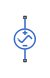# AC Voltage Source

Ideal constant voltage source

## Library

Electrical Sources

•## Description

The AC Voltage Source block represents an ideal voltage source that maintains sinusoidal voltage across its output terminals, independent of the current flowing through the source.

The output voltage is defined by the following equation:

`$V={V}_{0}\cdot \mathrm{sin}\left(2\pi \cdot f\cdot t+\phi \right)$`

where

 V Voltage V0 Peak amplitude f Frequency φ Phase shift t Time

Connections + and – are conserving electrical ports corresponding to the positive and negative terminals of the voltage source, respectively. The current is positive if it flows from positive to negative, and the voltage across the source is equal to the difference between the voltage at the positive and the negative terminal, V(+) – V(–).

Note

For Release 2012b and earlier, the unit definition for Hz was rev/s, whereas in R2013a it was changed to be 1/s, in compliance with the SI unit system. For this block it means that you must specify frequency in units of Hz or directly convertible to Hz, such as 1/s, kHz, MHz and GHz. In 2012b and earlier you could also specify frequency in angular units (such as rad/s or rpm), but this is no longer possible because the internal equation of the block now uses the 2π conversion factor to account for the 1/s unit definition. If you use this block in a model created prior to R2013a, update it by using the Upgrade Advisor. For more information, see the R2013a Release Notes.

## Parameters

Peak amplitude

Peak voltage amplitude. The default value is `1` V.

Phase shift

Phase shift in angular units. The default value is `0`.

Frequency

Voltage frequency, specified in Hz or units directly convertible to Hz (where Hz is defined as 1/s). For example, kHz and MHz are valid units, but rad/s is not. The default value is `60` Hz.

## Ports

The block has the following ports:

`+`

Electrical conserving port associated with the source positive terminal.

`–`

Electrical conserving port associated with the source negative terminal.

## Version History

Introduced in R2007a